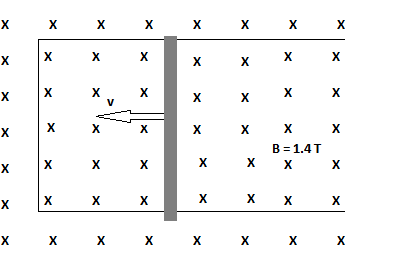# Problem: The figure shows a 21 cm long metal rod pulled along two frictionless, conducting rails at a constant speed of 3.0 m/s. The rails have negligible resistance but the rod has a resistance of 0.70 Ω. If the applied magnetic field is 1.4 T:a. What is the current induced in the rod?b. What is the magnitude of the force required to keep the rod moving at a constant speed?c. And what is the direction of this force?

###### FREE Expert Solution

Current induced in the rod:

$\overline{){\mathbf{i}}{\mathbf{=}}\frac{\mathbf{v}\mathbf{l}\mathbf{B}}{\mathbf{R}}}$

84% (489 ratings)###### Problem Details

The figure shows a 21 cm long metal rod pulled along two frictionless, conducting rails at a constant speed of 3.0 m/s. The rails have negligible resistance but the rod has a resistance of 0.70 ΩIf the applied magnetic field is 1.4 T:

a. What is the current induced in the rod?

b. What is the magnitude of the force required to keep the rod moving at a constant speed?

c. And what is the direction of this force?